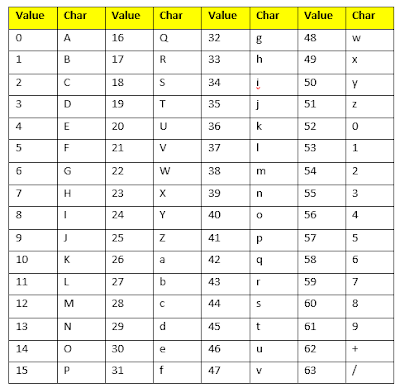# How Much Time Does Base64 Modules Takes To Encode or Decode an image in Python?

What is Base64?

Base64 is a type of conversion of bytes to ASCII(American Standard Code For Information Interchange) characters. When the computer converts  Base64 characters to Binary, each Base64 represents 6bits data of information. This is done using only the characters A-Z(upper letter), a-z(lower case letter), 0-9(number), + and /(for new line) in order to represent data.The = Character is used for padding at the end of the data stream.

Base64 Encoding?

Base64 is binary to ASCII Encoding scheme each base character represents 6 bits of data.

If we were to encode a string to Base64, we would follow these steps:

• Get the ASCII value of each character in the string.
• The 6-Bit Binary equivalent of the ASCII value.
• The large set of the binary number is divided into equal sections, each section containing 6bit.
• Convert the 6-bit binary group to their respective decimal value.
• Use the Base64 encoding table to align the respective Base64 value for each decimal value.Base64 Encoding Table

Base64 Decoding?

Base64 decoding is exactly reversed of the Base64 encoding process. If we were to decode Base64, we would follow these steps:
• Get the ASCII value of each character in the decimal value.
• The decimal value obtained is converted binary equivalent.
• The decimal value obtained is converted into their ASCII equivalent.
• The 8-bit binary number is converted to their decimal equivalents.

Convent Image to Base64 String in Python

To convert the image to the Base64 string in Python, we have used b64encode() method. Python base64.b64encode()function encode a string using Base64. Before exiting the function, we decode the bytes from base64.b64encode as a string.

This post first appeared on Stomenia, please read the originial post: here

# Share the post

How Much Time Does Base64 Modules Takes To Encode or Decode an image in Python?

×